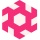Home
IT Knowledge
Inspiration
Languages
EN

# Python - math.e property example

0 points
Created by:Teilsa
345

The `Math.E` property returns e mathematical constant (`2.718281828459045...`).

`e` is called Euler's number or Napier's constant. However, it was discovered by Jacob Bernoulli. It is a mathematical constant used as the base of the natural logarithm.

``````import math

print(math.e)  # 2.718281828459045

print(math.exp(1))  # 2.718281828459045
print(math.exp(2))  # 7.38905609893065
print(math.exp(3))  # 20.085536923187668``````

## 1. Documentation

 Syntax ``math.e`` Result `e` number (`2.718281828459045...`). Description `math.e` constant returns the Euler's number.

## 2. `e` number approximation example

To calculate `e` the following function with infinity series can be used to get a better precision infinite number of iterations with big precision numbers.

``````import math

def calculateE(iterations):
return sum(1 / float(math.factorial(i)) for i in range(iterations))

print(calculateE(1))    # 1.0
print(calculateE(2))    # 2.0
print(calculateE(5))    # 2.708333333333333
print(calculateE(10))   # 2.7182815255731922
print(calculateE(20))   # 2.7182818284590455``````

## References

1. e (mathematical constant) - Wikipedia